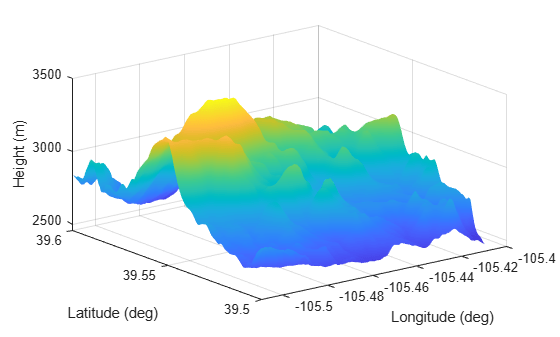# SurfaceManager

Since R2022a

## Description

The `SurfaceManager` object lists the surfaces in a radar scenario, `radarScenario`. You can enable or disable occlusion by surfaces in the radar scenario. The `occlusion` object function determines if any surfaces occlude the line of sight between two points. Use the `height` object function to query the height of surfaces at any location in the scenario.

## Creation

Use the `landSurface` object function to create `LandSurface` objects. Use `seaSurface` object function to create `SeaSurface` objects. Obtain the `SurfaceManager` object from the `SurfaceManager` property of the `radarScenario` object.

## Properties

expand all

Enable line-of-sight occlusion by surfaces, specified as `true` or `false`.

When specified as:

• `true` — The scenario models the occlusion of the line-of-sight by surfaces between points. In this case, the `detect` (Sensor Fusion and Tracking Toolbox) object function of the `radarScenario` object or the `detect` (Sensor Fusion and Tracking Toolbox) object function of the `Platform` (Sensor Fusion and Tracking Toolbox) object accounts for surface occlusion.

Note

If the `IsEarthCentered` property of the `radarScenario` object is specified as `true`, selecting this option also enables horizon occlusion based on the WGS84 Earth model.

• `false` — The scenario does not models the occlusion of the line-of-sight due to ground surfaces or the WGS84 Earth model.

Surfaces in radar scenario, specified as an array of `LandSurface` and `SeaSurface` objects.

You can add land surfaces to a radar scenario using the `landSurface` and sea surfaces using the `seaSurface` object function.

## Object Functions

 `height` Height of point on surface `occlusion` Test for occlusion of point by a surface

## Examples

collapse all

Create a radar scenario and specify its `IsEarthCentered` property as `true to use DTED file`.

`scene = radarScenario(IsEarthCentered = true);`

Model the reflectivity as a constant gamma surface.

`refl = surfaceReflectivityLand(Model = 'ConstantGamma',Gamma = -20);`

Add a 0.1-by-0.1 degree land surface derived from a DTED file.

```bdry = [39.5 39.6;-105.51 -105.41]; srf = landSurface(scene,Terrain = 'n39_w106_3arc_v2.dt1', ... Boundary = bdry,RadarReflectivity = refl)```
```srf = LandSurface with properties: RadarReflectivity: [1x1 surfaceReflectivityLand] ReflectivityMap: 1 ReferenceHeight: 0 Boundary: [2x2 double] Terrain: 'n39_w106_3arc_v2.dt1' ```
`mgr = scene.SurfaceManager`
```mgr = SurfaceManager with properties: UseOcclusion: 1 Surfaces: [1x1 radar.scenario.LandSurface] ```

Plot the surface height.

```x = linspace(srf.Boundary(2,1),srf.Boundary(2,2),201); y = linspace(srf.Boundary(1,1),srf.Boundary(1,2),201); [X,Y] = meshgrid(x,y); X1 = X(:)'; Y1 = Y(:)'; H = height(srf,[Y1;X1]); H = reshape(H,length(x),length(y)); surf(x,y,H) shading interp ylabel('Latitude (deg)') xlabel('Longitude (deg)') zlabel('Height (m)')```## Version History

Introduced in R2022a